• 2021-03-24 01:48:04

这里的合成是多张图片合成在一张里，不是合成为GIF图片，需要的朋友可以直接下载使用。

图片合成，可以显示在浏览器上面同时保存到文件夹下面

实例如下所示：<?php

/**

* 图片合并

**/

$pic_list = array( 'img2.png', 'img2.png', 'logo.png', 'logo.png', 'logo.png', 'img2.png', 'img2.png', 'img2.png', 'img2.png' );$pic_list = array_slice($pic_list, 0, 9); // 只操作前9个图片$bg_w = 150; // 背景图片宽度

$bg_h = 150; // 背景图片高度$background = imagecreatetruecolor($bg_w,$bg_h); // 背景图片

$color = imagecolorallocate($background, 202, 201, 201); // 为真彩色画布创建白色背景，再设置为透明

imagefill($background, 0, 0,$color);

imageColorTransparent($background,$color);

$pic_count = count($pic_list);

$lineArr = array(); // 需要换行的位置$space_x = 3;

$space_y = 3;$line_x = 0;

switch($pic_count) { case 1: // 正中间$start_x = intval($bg_w/4); // 开始位置X$start_y = intval($bg_h/4); // 开始位置Y$pic_w = intval($bg_w/2); // 宽度$pic_h = intval($bg_h/2); // 高度 break; case 2: // 中间位置并排$start_x = 2;

$start_y = intval($bg_h/4) + 3;

$pic_w = intval($bg_w/2) - 5;

$pic_h = intval($bg_h/2) - 5;

$space_x = 5; break; case 3:$start_x = 40; // 开始位置X

$start_y = 5; // 开始位置Y$pic_w = intval($bg_w/2) - 5; // 宽度$pic_h = intval($bg_h/2) - 5; // 高度$lineArr = array(2);

$line_x = 4; break; case 4:$start_x = 4; // 开始位置X

$start_y = 5; // 开始位置Y$pic_w = intval($bg_w/2) - 5; // 宽度$pic_h = intval($bg_h/2) - 5; // 高度$lineArr = array(3);

$line_x = 4; break; case 5:$start_x = 30; // 开始位置X

$start_y = 30; // 开始位置Y$pic_w = intval($bg_w/3) - 5; // 宽度$pic_h = intval($bg_h/3) - 5; // 高度$lineArr = array(3);

$line_x = 5; break; case 6:$start_x = 5; // 开始位置X

$start_y = 30; // 开始位置Y$pic_w = intval($bg_w/3) - 5; // 宽度$pic_h = intval($bg_h/3) - 5; // 高度$lineArr = array(4);

$line_x = 5; break; case 7:$start_x = 53; // 开始位置X

$start_y = 5; // 开始位置Y$pic_w = intval($bg_w/3) - 5; // 宽度$pic_h = intval($bg_h/3) - 5; // 高度$lineArr = array(2,5);

$line_x = 5; break; case 8:$start_x = 30; // 开始位置X

$start_y = 5; // 开始位置Y$pic_w = intval($bg_w/3) - 5; // 宽度$pic_h = intval($bg_h/3) - 5; // 高度$lineArr = array(3,6);

$line_x = 5; break; case 9:$start_x = 5; // 开始位置X

$start_y = 5; // 开始位置Y$pic_w = intval($bg_w/3) - 5; // 宽度$pic_h = intval($bg_h/3) - 5; // 高度$lineArr = array(4,7);

$line_x = 5; break; } foreach($pic_list as $k=>$pic_path ) {

$kk =$k + 1;

if ( in_array($kk,$lineArr) ) {

$start_x =$line_x;

$start_y =$start_y + $pic_h +$space_y;

}

$pathInfo = pathinfo($pic_path);

switch( strtolower($pathInfo['extension']) ) { case 'jpg': case 'jpeg':$imagecreatefromjpeg = 'imagecreatefromjpeg';

break;

case 'png':

$imagecreatefromjpeg = 'imagecreatefrompng'; break; case 'gif': default:$imagecreatefromjpeg = 'imagecreatefromstring';

$pic_path = file_get_contents($pic_path);

break;

}

$resource =$imagecreatefromjpeg($pic_path); //$start_x,$start_y copy图片在背景中的位置 // 0,0 被copy图片的位置 //$pic_w,$pic_h copy后的高度和宽度 imagecopyresized($background,$resource,$start_x,$start_y,0,0,$pic_w,$pic_h,imagesx($resource),imagesy($resource)); // 最后两个参数为原始图片宽度和高度，倒数两个参数为copy时的图片宽度和高度$start_x = $start_x +$pic_w + $space_x; } header("Content-type: image/jpg"); imagejpeg($background);

imagegif($background, "./hero_gam.png"); ?> 相信看了这些案例你已经掌握了方法，更多精彩请关注php中文网其它相关文章！ 相关阅读： 更多相关内容 • 包含icepdf-core-4.3.3.jar包及后端代码，将多张PDF转为一张图片，水印、图章无法转出，更改代码中文件路径，加入JAR包运行即可。 • 1.多张图片合成一张比如：图片合成，可以显示在浏览器上面同时保存到文件夹下面实例如下所示：/*** 图片合并**/$pic_list = array('img2.png','img2.png','logo.png','logo.png','logo.png','im...

PHP如何进行图片处理呢？是否可以将多张图片合成一张呢？本文就以PHP将多张图片合成一张，来让大家对PHP的图片处理有更清晰的认识。

1.多张图片合成一张比如：

图片合成，可以显示在浏览器上面同时保存到文件夹下面实例如下所示：

/**

* 图片合并

**/

$pic_list = array( 'img2.png', 'img2.png', 'logo.png', 'logo.png', 'logo.png', 'img2.png', 'img2.png', 'img2.png', 'img2.png' );$pic_list = array_slice($pic_list, 0, 9); // 只操作前9个图片$bg_w = 150; // 背景图片宽度

$bg_h = 150; // 背景图片高度$background = imagecreatetruecolor($bg_w,$bg_h); // 背景图片

$color = imagecolorallocate($background, 202, 201, 201); // 为真彩色画布创建白色背景，再设置为透明

imagefill($background, 0, 0,$color);

imageColorTransparent($background,$color);

$pic_count = count($pic_list);

$lineArr = array(); // 需要换行的位置$space_x = 3;

$space_y = 3;$line_x = 0;

switch($pic_count) { case 1: // 正中间$start_x = intval($bg_w/4); // 开始位置X$start_y = intval($bg_h/4); // 开始位置Y$pic_w = intval($bg_w/2); // 宽度$pic_h = intval($bg_h/2); // 高度 break; case 2: // 中间位置并排$start_x = 2;

$start_y = intval($bg_h/4) + 3;

$pic_w = intval($bg_w/2) - 5;

$pic_h = intval($bg_h/2) - 5;

$space_x = 5; break; case 3:$start_x = 40; // 开始位置X

$start_y = 5; // 开始位置Y$pic_w = intval($bg_w/2) - 5; // 宽度$pic_h = intval($bg_h/2) - 5; // 高度$lineArr = array(2);

$line_x = 4; break; case 4:$start_x = 4; // 开始位置X

$start_y = 5; // 开始位置Y$pic_w = intval($bg_w/2) - 5; // 宽度$pic_h = intval($bg_h/2) - 5; // 高度$lineArr = array(3);

$line_x = 4; break; case 5:$start_x = 30; // 开始位置X

$start_y = 30; // 开始位置Y$pic_w = intval($bg_w/3) - 5; // 宽度$pic_h = intval($bg_h/3) - 5; // 高度$lineArr = array(3);

$line_x = 5; break; case 6:$start_x = 5; // 开始位置X

$start_y = 30; // 开始位置Y$pic_w = intval($bg_w/3) - 5; // 宽度$pic_h = intval($bg_h/3) - 5; // 高度$lineArr = array(4);

$line_x = 5; break; case 7:$start_x = 53; // 开始位置X

$start_y = 5; // 开始位置Y$pic_w = intval($bg_w/3) - 5; // 宽度$pic_h = intval($bg_h/3) - 5; // 高度$lineArr = array(2,5);

$line_x = 5; break; case 8:$start_x = 30; // 开始位置X

$start_y = 5; // 开始位置Y$pic_w = intval($bg_w/3) - 5; // 宽度$pic_h = intval($bg_h/3) - 5; // 高度$lineArr = array(3,6);

$line_x = 5; break; case 9:$start_x = 5; // 开始位置X

$start_y = 5; // 开始位置Y$pic_w = intval($bg_w/3) - 5; // 宽度$pic_h = intval($bg_h/3) - 5; // 高度$lineArr = array(4,7);

$line_x = 5; break; } foreach($pic_list as $k=>$pic_path ) {

$kk =$k + 1;

if ( in_array($kk,$lineArr) ) {

$start_x =$line_x;

$start_y =$start_y + $pic_h +$space_y;

}

$pathInfo = pathinfo($pic_path);

switch( strtolower($pathInfo['extension']) ) { case 'jpg': case 'jpeg':$imagecreatefromjpeg = 'imagecreatefromjpeg';

break;

case 'png':

$imagecreatefromjpeg = 'imagecreatefrompng'; break; case 'gif': default:$imagecreatefromjpeg = 'imagecreatefromstring';

$pic_path = file_get_contents($pic_path);

break;

}

$resource =$imagecreatefromjpeg($pic_path); //$start_x,$start_y copy图片在背景中的位置 // 0,0 被copy图片的位置 //$pic_w,$pic_h copy后的高度和宽度 imagecopyresized($background,$resource,$start_x,$start_y,0,0,$pic_w,$pic_h,imagesx($resource),imagesy($resource)); // 最后两个参数为原始图片宽度和高度，倒数两个参数为copy时的图片宽度和高度$start_x = $start_x +$pic_w + $space_x; } header("Content-type: image/jpg"); imagejpeg($background);

imagegif($background, "./hero_gam.png"); ?> 相关推荐： PHP GD 图像处理组件的常用函数总结 php 图片操作类,支持生成缩略图,添加水印,上传缩略图 很强的 PHP 图片处理类 展开全文• 特效描述：利用JS实现 多张图片合成 一张图片代码。利用JS实现多张图片合成一张图片代码代码结构1. HTML代码注：请在服务端运行才能看到效果 function hecheng(){draw(function(){document.getElementById('imgBox')... 特效描述：利用JS实现 多张图片合成 一张图片代码。利用JS实现多张图片合成一张图片代码 代码结构 1. HTML代码 ### 注：请在服务端运行才能看到效果function hecheng(){ draw(function(){ document.getElementById('imgBox').innerHTML=' 合成图片成功！可以鼠标另存图片查看我是否是一张图片~~！'; }) } var data=['img1.png','img2.png','img3.png','img4.png'],base64=[]; function draw(fn){ var c=document.createElement('canvas'), ctx=c.getContext('2d'), len=data.length; c.width=290; c.height=290; ctx.rect(0,0,c.width,c.height); ctx.fillStyle='#fff'; ctx.fill(); function drawing(n){ if(n var img=new Image; //img.crossOrigin = 'Anonymous'; //解决跨域 img.src=data[n]; img.οnlοad=function(){ ctx.drawImage(img,0,0,290,290); drawing(n+1);//递归 } }else{ //保存生成作品图片 base64.push(c.toDataURL("image/jpeg",0.8)); //alert(JSON.stringify(base64)); fn(); } } drawing(0); } 展开全文• 首先，我们需要把每天的每个小时图片，切割成一张图片，再把24个小时拼接成一个完整的图片 原： 切割： 切割图片，请看https://blog.csdn.net/qq_16855077/article/details/90378173 ... # 1.准备 场景：可能会遇到这样的需求，让我们查看一天每个小时的变化情况，并在一张图片中体现出来，那这就需要利用图片的切割和合成技术。 首先，我们需要把每天的每个小时图片，切割成一张图片，再把24个小时拼接成一个完整的图片 原图：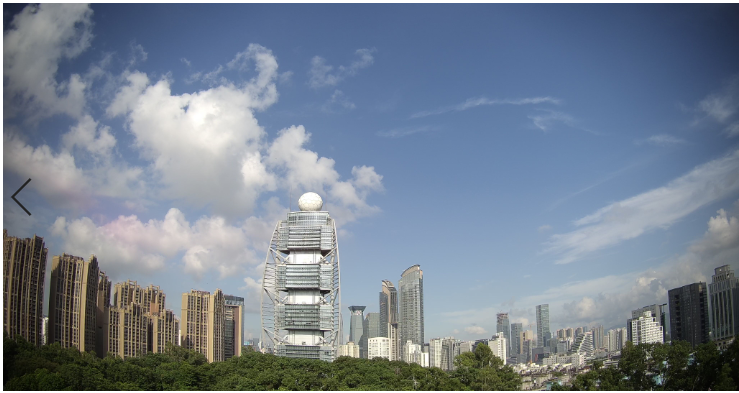切割图：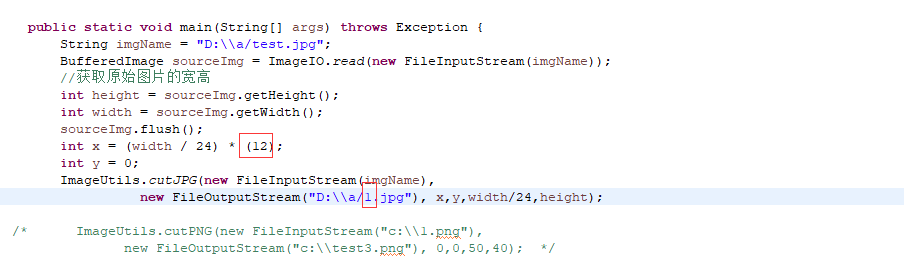代码的这两个地方改为12 1.jpg 13 2.jpg 14 3.jpg，生成的图片如下图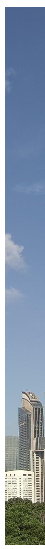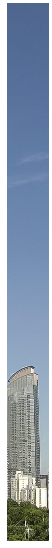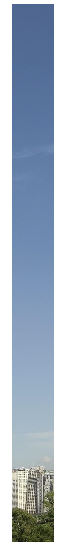合成图：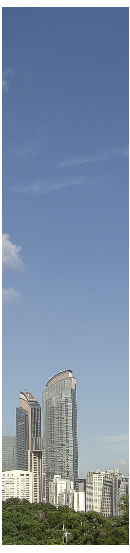# 2.代码 package com.qihui.qxj.utils; import java.awt.image.BufferedImage; import java.io.File; import javax.imageio.ImageIO; /** * 图片拼接 把多张宽度一样的图片拼接成一张大图片 * * @author Administrator * */ public class CreateBigImage { public static void main(String[] args) { // 获取宽度相同的图片 String img1 = "D:/a/1.jpg"; String img2 = "D:/a/2.jpg"; String img3 = "D:/a/3.jpg"; String[] imgs = new String[] { img1, img2, img3 }; //Image.mergeImage(imgs, 1, "D:/big.jpg"); // //图片拼接 merge(imgs, "jpg", "D:/big.jpg"); } /** * Java拼接多张图片 * * @param pics:图片源文件 * （必须要宽度一样），如： String img1 = "D:/imgs/3.jpg"; String img2 = * "D:/imgs/3.jpg"; String img3 = "D:/imgs/big.jpg"; String[] * pics = new String[]{img1,img2,img3}; * @param type * ：图片输出类型（jpg，png，jpeg...） * @param dst_pic * ：图片输出绝对路径，如 String dst_pic="D:/imgs/big2.jpg"; * @return */ public static boolean merge(String[] pics, String type, String dst_pic) { int len = pics.length; // 图片文件个数 if (len < 1) { System.out.println("pics len < 1"); return false; } File[] src = new File[len]; BufferedImage[] images = new BufferedImage[len]; int[][] ImageArrays = new int[len][]; for (int i = 0; i < len; i++) { try { src[i] = new File(pics[i]); images[i] = ImageIO.read(src[i]); } catch (Exception e) { e.printStackTrace(); return false; } int width = images[i].getWidth(); int height = images[i].getHeight(); ImageArrays[i] = new int[width * height];// 从图片中读取RGB ImageArrays[i] = images[i].getRGB(0, 0, width, height, ImageArrays[i], 0, width); } int dst_width = 0; int dst_height = 0; for (int i = 0; i < images.length; i++) { dst_width += images[i].getWidth(); dst_height = dst_height > images[i].getHeight() ? dst_height : images[i].getHeight(); } System.out.println(dst_width); System.out.println(dst_height); // 生成新图片 try { BufferedImage ImageNew = new BufferedImage(dst_width, dst_height, BufferedImage.TYPE_INT_RGB); int width_i = 0; for (int i = 0; i < images.length; i++) { ImageNew.setRGB(width_i, 0, images[i].getWidth(), dst_height, ImageArrays[i], 0, images[i].getWidth()); width_i += images[i].getWidth(); } File outFile = new File(dst_pic); ImageIO.write(ImageNew, type, outFile);// 写图片 } catch (Exception e) { e.printStackTrace(); return false; } return true; } } 到这里，图片的合成就完成勒。 如果你热衷技术，喜欢交流，欢迎加入我们！展开全文ffmpeg • 应客户要求 需要把两张图片合成一张保存起来 一张背景 一张二维码 用于二维码扫描 区分代理和上下级关系 期望の效果 实现流程 背景 客户希望是自己可以上传 这样不那么单调 我们从后台接口回去imageUrl 转成...二维码生成 QRCode • 如何利用qt合成图片，本例主要利用两张图片进行合成一张 • 那么，当我们遇到要将多张图片合成一个PDF文件的情况，我们该怎么办呢？今天，小编就教各位将多张图片合成一个PDF文件的小技巧，大家一起来学习吧！首先，我们打开WPS软件，切换到【PDF】页面；然后，我们按左键单击... • 主要介绍了Android开发之将两张图片合并为一张图片的方法,涉及Android基于Canvas类操作图片的相关技巧,需要的朋友可以参考下 • 1.多张图片合成一张比如：图片合成，可以显示在浏览器上面同时保存到文件夹下面实例如下所示： • }, //多图合一 drawAndShareImage() { var canvas = document.createElement("canvas"); canvas.width = 850; canvas.height = 400; var context = canvas.getContext("2d"); ... • C# 使用EmguCV 拼接图片（用于照片合成全景） ，一个简单的应用程序，选择几张图片，拼接图片，并保存 • 单一的静态图片太枯燥，GIF动态图片看起来才有意思有木有！网上各种经典爆笑GIF图片或者闪图，大多数的友友们只会看→复制→外发，...今天我们来学第二种：把多张图片合成gif动态图片。跟着小编的步骤一起制作：g... • 在网络上我们经常见到的电子相册其本质就是图片音乐合成视频，使用一些图片合成视频软件将多张图片合成视频，外加点炫酷的转场特效，so easy的就能完成了。o(*≧▽≦)ツ 想不想知道具体的操作过程？有兴趣的童鞋可以... • https://blog.csdn.net/wangshuainan/article/details/77914508cat Z000*.jpg | ffmpeg -r 3 -t 12 -i pipe:0 -y -b 200K out.mp4FFmpeg将多张图片合成视频首先要计算出视频的总帧数：总帧数 = duration * fps 。... • 需求说明：制作一个马赛克图片，将几万张图片进行合成，每个马赛克格子的尺寸为15x15。 完整源码在文末有说明，需要的可以去下载哈。 【阅读全文】 看一下生成的马赛克图片的效果： 需要使用到的python模块包如下...python 笔记 python 技巧 趣味python • 伊谢尔伦2017-04-10 17:27:582楼$imgs = array();$imgs = 'imgs/1.jpg';$imgs = 'imgs/2.jpg';$imgs = 'imgs/3.jpg';$imgs = 'imgs/4.jpg';... //背景图片\$target_img = Imagecreatefromjpe...
• 使用codeplex的GifCreator来操作 步骤： 一 获取动态链接库Gif.Components.dll ...二 新建一个winfom程序，...注意：转换耗时很，建议开一个新的线程,我就5张图片合成一张gif却耗时了5秒，不是我想要的效果C#
• 小编作为一个上班族，每天会涉及到很的图片文件，日积月累图片文件的整理和保存是一个任务艰巨的工作...小编在日常工作中总结了两种方法这个问题，接下来就由我来为大家详细介绍两种方法将多张图片怎么合成一个pd...
• java将多张图片合成视频 需求 近几天，无聊就看看抖音，视频信息传播信息，相亲去抖音，网红去抖音，秀恩爱去抖音。。。走在大街上，几个小妹妹拿着手机自拍干是玩抖音还是直播呢？每个人都想当导演的梦，但又没DV...
• 效果如下 效果如下 项目结构如下 项目中需要引入Gif.Components.dll插件，可从文章顶部链接免费下载， 也可从网盘直接下载： 网盘地址：https://pan.baidu.com/s/1nko3jbK6dsAedBz1DHG92A 提取码...c#
• 首先要收集若干张图片，然后裁切成1:1的正方形，若干张张都要裁切成1:1。 单一图片裁剪方法： 文件——>打开——>选择图片——>选择左侧的裁剪工具——>在上侧选择1:1(方形)——>√ 2.2photoshop
• package com.yunfengtech.solution.business; import java.awt.Color; import java.awt.Graphics; import java.awt.image.BufferedImage; import java.io.File;...import java.io.FileInputStream;...import java.io.F...java
• 将两张图片多张图片进行合并，并在本地生成一张新的图片
• 多张图片合成一张,不说直接上代码：注意当图片宽度不同时会报数组下标异常。 /** * *@类功能说明:java拼接多张图片，生成的格式是jpg、bmp、gif等， * 如果其他格式则报错或者photoshop或者画图工具打不...
• 在平常我们开发的时候, 我们会遇到各种各样的需求, 比如想微博那样, 把多张图片合成一张长图, 现在让我们来看看怎么把多张图片合成一张长图. 1.布置界面 2.实现代码一(绘制多张图片) // 1.把张绘制成一...Image
• 现如今制作gif图片也不是什么难事了，巧用动态图合成软件，即能轻松将多张静态图片合成一张动态。动态图片是由多张不同的静态图片组合而成的gif格式图片，它会按照一定的顺序和时间进行逐帧播放。做好的动态，...
• uni-app通过canvas将两张图片合成一张图片 <template> <view class="share"> <button type="default" @click="exportPost"></button> <view id="pre_style"> //预览合成的...vue
• from PIL import Imagefrom os import listdir'''result_path_target 图片存放目标路径cut_pictures 待拼接图片存放路径num 图片命名以数字按序增加'''cut_pictures = r'E:\照片'result_path_target = r'E:\照片\1'......